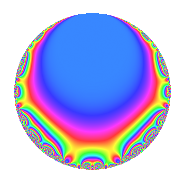# Properties

 Label 62.2.a.bLevel 62 Weight 2 Character orbit 62.a Self dual Yes Analytic conductor 0.495 Analytic rank 0 Dimension 2 CM No Inner twists 1

# Related objects

## Newspace parameters

 Level: $$N$$ = $$62 = 2 \cdot 31$$ Weight: $$k$$ = $$2$$ Character orbit: $$[\chi]$$ = 62.a (trivial)

## Newform invariants

 Self dual: Yes Analytic conductor: $$0.495072492532$$ Analytic rank: $$0$$ Dimension: $$2$$ Coefficient field: $$\Q(\sqrt{3})$$ Coefficient ring: $$\Z[a_1, a_2, a_3]$$ Coefficient ring index: $$1$$ Fricke sign: $$-1$$ Sato-Tate group: $\mathrm{SU}(2)$

## $q$-expansion

Coefficients of the $$q$$-expansion are expressed in terms of $$\beta = \sqrt{3}$$. We also show the integral $$q$$-expansion of the trace form.

 $$f(q)$$ $$=$$ $$q - q^{2} + ( 1 + \beta ) q^{3} + q^{4} -2 \beta q^{5} + ( -1 - \beta ) q^{6} + 2 q^{7} - q^{8} + ( 1 + 2 \beta ) q^{9} +O(q^{10})$$ $$q - q^{2} + ( 1 + \beta ) q^{3} + q^{4} -2 \beta q^{5} + ( -1 - \beta ) q^{6} + 2 q^{7} - q^{8} + ( 1 + 2 \beta ) q^{9} + 2 \beta q^{10} + ( -3 + \beta ) q^{11} + ( 1 + \beta ) q^{12} + ( -1 - 3 \beta ) q^{13} -2 q^{14} + ( -6 - 2 \beta ) q^{15} + q^{16} + 2 \beta q^{17} + ( -1 - 2 \beta ) q^{18} -4 q^{19} -2 \beta q^{20} + ( 2 + 2 \beta ) q^{21} + ( 3 - \beta ) q^{22} + ( -1 - \beta ) q^{24} + 7 q^{25} + ( 1 + 3 \beta ) q^{26} + 4 q^{27} + 2 q^{28} + ( -3 + 3 \beta ) q^{29} + ( 6 + 2 \beta ) q^{30} + q^{31} - q^{32} -2 \beta q^{33} -2 \beta q^{34} -4 \beta q^{35} + ( 1 + 2 \beta ) q^{36} + ( 5 + 3 \beta ) q^{37} + 4 q^{38} + ( -10 - 4 \beta ) q^{39} + 2 \beta q^{40} + ( 6 - 2 \beta ) q^{41} + ( -2 - 2 \beta ) q^{42} + ( -1 + 3 \beta ) q^{43} + ( -3 + \beta ) q^{44} + ( -12 - 2 \beta ) q^{45} + 6 q^{47} + ( 1 + \beta ) q^{48} -3 q^{49} -7 q^{50} + ( 6 + 2 \beta ) q^{51} + ( -1 - 3 \beta ) q^{52} + ( 3 + \beta ) q^{53} -4 q^{54} + ( -6 + 6 \beta ) q^{55} -2 q^{56} + ( -4 - 4 \beta ) q^{57} + ( 3 - 3 \beta ) q^{58} + ( -6 + 2 \beta ) q^{59} + ( -6 - 2 \beta ) q^{60} + ( -1 - 3 \beta ) q^{61} - q^{62} + ( 2 + 4 \beta ) q^{63} + q^{64} + ( 18 + 2 \beta ) q^{65} + 2 \beta q^{66} + 8 q^{67} + 2 \beta q^{68} + 4 \beta q^{70} -8 \beta q^{71} + ( -1 - 2 \beta ) q^{72} -10 q^{73} + ( -5 - 3 \beta ) q^{74} + ( 7 + 7 \beta ) q^{75} -4 q^{76} + ( -6 + 2 \beta ) q^{77} + ( 10 + 4 \beta ) q^{78} + ( 2 - 6 \beta ) q^{79} -2 \beta q^{80} + ( 1 - 2 \beta ) q^{81} + ( -6 + 2 \beta ) q^{82} + ( 3 - 5 \beta ) q^{83} + ( 2 + 2 \beta ) q^{84} -12 q^{85} + ( 1 - 3 \beta ) q^{86} + 6 q^{87} + ( 3 - \beta ) q^{88} + 6 q^{89} + ( 12 + 2 \beta ) q^{90} + ( -2 - 6 \beta ) q^{91} + ( 1 + \beta ) q^{93} -6 q^{94} + 8 \beta q^{95} + ( -1 - \beta ) q^{96} + ( 2 + 6 \beta ) q^{97} + 3 q^{98} + ( 3 - 5 \beta ) q^{99} +O(q^{100})$$ $$\operatorname{Tr}(f)(q)$$ $$=$$ $$2q - 2q^{2} + 2q^{3} + 2q^{4} - 2q^{6} + 4q^{7} - 2q^{8} + 2q^{9} + O(q^{10})$$ $$2q - 2q^{2} + 2q^{3} + 2q^{4} - 2q^{6} + 4q^{7} - 2q^{8} + 2q^{9} - 6q^{11} + 2q^{12} - 2q^{13} - 4q^{14} - 12q^{15} + 2q^{16} - 2q^{18} - 8q^{19} + 4q^{21} + 6q^{22} - 2q^{24} + 14q^{25} + 2q^{26} + 8q^{27} + 4q^{28} - 6q^{29} + 12q^{30} + 2q^{31} - 2q^{32} + 2q^{36} + 10q^{37} + 8q^{38} - 20q^{39} + 12q^{41} - 4q^{42} - 2q^{43} - 6q^{44} - 24q^{45} + 12q^{47} + 2q^{48} - 6q^{49} - 14q^{50} + 12q^{51} - 2q^{52} + 6q^{53} - 8q^{54} - 12q^{55} - 4q^{56} - 8q^{57} + 6q^{58} - 12q^{59} - 12q^{60} - 2q^{61} - 2q^{62} + 4q^{63} + 2q^{64} + 36q^{65} + 16q^{67} - 2q^{72} - 20q^{73} - 10q^{74} + 14q^{75} - 8q^{76} - 12q^{77} + 20q^{78} + 4q^{79} + 2q^{81} - 12q^{82} + 6q^{83} + 4q^{84} - 24q^{85} + 2q^{86} + 12q^{87} + 6q^{88} + 12q^{89} + 24q^{90} - 4q^{91} + 2q^{93} - 12q^{94} - 2q^{96} + 4q^{97} + 6q^{98} + 6q^{99} + O(q^{100})$$

## Embeddings

For each embedding $$\iota_m$$ of the coefficient field, the values $$\iota_m(a_n)$$ are shown below.

For more information on an embedded modular form you can click on its label.

Label $$\iota_m(\nu)$$ $$a_{2}$$ $$a_{3}$$ $$a_{4}$$ $$a_{5}$$ $$a_{6}$$ $$a_{7}$$ $$a_{8}$$ $$a_{9}$$ $$a_{10}$$
1.1
 −1.73205 1.73205
−1.00000 −0.732051 1.00000 3.46410 0.732051 2.00000 −1.00000 −2.46410 −3.46410
1.2 −1.00000 2.73205 1.00000 −3.46410 −2.73205 2.00000 −1.00000 4.46410 3.46410
 $$n$$: e.g. 2-40 or 990-1000 Significant digits: Format: Complex embeddings Normalized embeddings Satake parameters Satake angles

## Inner twists

This newform does not admit any (nontrivial) inner twists.

## Atkin-Lehner signs

$$p$$ Sign
$$2$$ $$1$$
$$31$$ $$-1$$

## Hecke kernels

This newform can be constructed as the kernel of the linear operator $$T_{3}^{2} - 2 T_{3} - 2$$ acting on $$S_{2}^{\mathrm{new}}(\Gamma_0(62))$$.